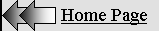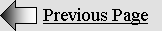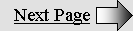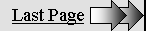KINETIC ENERGY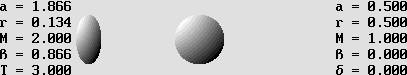The moving billiard ball transfers all of its kinetic energy to the stationary one.

This is possible because reciprocal active and reactive mass (a, r) is exchanged through the intermediate field of force.

Lorentz discovered that moving matter contains additional mass as kinetic energy, which is given by:

E  = (gamma 1) m c 2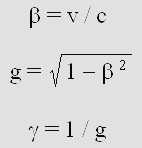The Newtonian formula below proves to be inaccurate because Newton was unaware of this additional mass.

E = m v 2 / 2

However, thanks to Lorentz's discovery, I could solve this problem.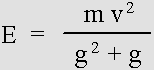Now, the Newtonian formula is perfectly accurate at any speed.

It turns out that Newton's mechanics is easily upgradeable. It is still the best choice.

UPGRADING NEWTON'S KINETIC ENERGY

Matter mechanics is highly dependant on kinetic energy, which is the consequence of motion. In the absence of motion, there would be no mechanics. Because it is a measure for a given motion inside a given space, the time itself would become meaningless.

In addition, matter is made of waves and all forces are caused by waves. Thus, matter mechanics should be called the wave mechanics, albeit it is more specifically the fields of force mechanics.

And finally, waves plus motion obviously lead to the Doppler effect. This web site has demonstrated a long time ago that the Lorentz Transformations are just a special Doppler effect which applies to the electron. This is why it becomes more and more powerful at very high speed.

This page explains why and how kinetic energy is related to this additional energy. Then it will become clear that Newton's laws are preferable to Einstein's mechanics because they are well known and much more understandable. However, they need some adjustment in order to hold perfectly true.

The field of force contains pure kinetic energy.

Two bags filled with sand cannot produce an elastic collision. Some of the energy is rather converted into heat, making the final result very hard to analyze. So let's take a better example. Suppose that one billiard ball hits another billiard ball head-on .

In this case, the repulsive force is that of the electrons, which behave like springs because of the Coulomb force. There are billions of them and they come very close together when both surfaces collide. At this moment, the electrostatic field, which is made of standing waves, contains a maximum of energy. It is potential energy because it will be transferred to the other ball.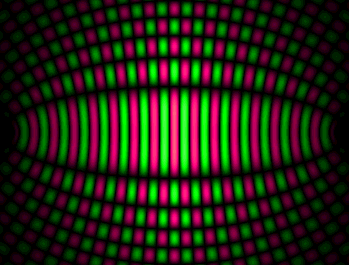The electrostatic field of force.

It is made out of traveling waves incoming from two electrons or positrons.

This produces standing waves, which are amplified afterwards by all other waves in the vicinity.

It is a well known fact that standing waves contain energy. Here, the field of force is only a temporary one. It is made out of waves emitted by electrons.

I showed in the page on standing waves that in case of motion, standing waves transform into "moving standing waves". In this case, nodes and antinodes are still present but they are moving according to the forward vs. backward Doppler ratio. Then the energy is transferred at the same speed, which is always slower than the speed of light.

That is why the field of force acts just like a spring. All of its potential energy, hence mass, was borrowed from the first ball and it is transferred to the second one.

The energy calculus.

Newton was totally unaware of this process. Surely, the Newtonian formula for kinetic energy below needs a severe upgrade.

E  =  m v 2 / 2

In this formula, E stands for energy in joules and m for mass in kilograms. Distances being in meters, v stands for matter speed and c for the speed of light in meters per second. This equation was forged by Voltaire's wife, Emilie Du Chatelet. However, the calculus leading to this achievement originated most certainly from Leibniz, who also created the differential calculus independently from Newton. It can easily be derived from Newton's two main equations on acceleration, especially f = m*a.

The Newtonian formula contains two errors.

It was discovered more than a century ago that this formula becomes inaccurate at very high "relativistic" speed. Lorentz found that mass should increase according to the gamma factor. This should be considered as one of the Lorentz transformations because matter contracts and clocks slow down according to the same "g" ratio.

Below, capital M stands for mass while matter is moving very fast. The mass gain is added to the mass at rest and it can easily be obtained using Lorentz's gamma factor: gamma = 1/g.

M  = gamma * m

Today, it is well known that mass is a measure of matter inertia and also a measure of its energy. The total mass M increases according to the gamma factor. It becomes theoretically infinite at the speed of light so that any speed faster than the speed of light is impossible. For instance, a billiard ball whose mass at rest was 1 kg transforms into a 2 kg ball and it becomes two times shorter (only on the x axis) at 86.6% of the speed of light according to the gamma factor (gamma = 2; g = 0.5).

The spherical billiard ball shown above on the right hand side is accelerated to 0.866 c and it is submitted to the Lorentz transformations. It becomes a squashed ellipsoid 50% shorter and its mass is doubled to 2 kg.

Because its inertia is also doubled, the ball cannot be accelerated again to 2 * 0.866 = 1.732 c using the same amount of energy. Additionally, this speed is much faster than the speed of light. Adding another kilogram of energy would rather increase its total mass M from 2 to 3 kg. Then gamma = 3, g = 1/3 and the new beta normalized speed is given by:

beta = Sqr(1 g 2 )

Thus, if the billiard ball was accelerated again using the same amount of energy, it would accelerate from 0.866 to 0.9428 c.

Let's take an example.

The example below should be useful in order to understand better the action and reaction process.

Beta normalized speed:  beta  =  v / c  =  sin 60°  =  (1 – g 2) 1 / 2  =  0.866

Speed of light:  c  =  300 000 000 m/s  (accurate: 299 792 458 m/s).

Matter velocity:  v  =  beta * c  =  259 807 621 m/s

Gamma factor:  gamma  =  1 / (1 – beta 2) 1 / 2  =  1 / g  =  2

Lorentz's shrinking factor:  g  =  (1 – beta 2) 1 / 2  =  1 / gamma  =  0.5

Stationary intrinsic mass:  m  =  1 kg

Total mass for fast moving matter:  M  =  gamma * m  =  2 kg

Mass gain:  G  =  gamma * m m  =  (gamma 1) m  =  1 kg

Stationary intrinsic mass energy :  E  =  m c 2  =  c 2  =  9 * 10 16  joules.

1 The mass gain for fast moving matter must be taken into account.

The first step is to modify the Newtonian formula for kinetic energy in such a way that the mass gain is taken into account. This is obtained quite simply by applying the gamma factor:

E  =  m v 2 / 2   is replaced by:  E  =  gamma * m v 2 / 2

However, this new formula still yields incorrect results. Poincare (followed by Einstein) rather elaborated this equation:

E  =  (gamma * m m) c 2   or:  E  =  (gamma 1) m c 2

This formula proves to be perfectly accurate. The gain in mass is indeed given by: G  =  (gamma 1) m  =  1

So that:  E  =  G c 2  =  c 2  =  9 * 10 16  joules.

Here, the speed was carefully chosen in order to obtain kinetic energy which equals the mass at rest: exactly one kilogram. The next step will be to explain why the modified Newtonian formula elaborated above still does not work. It seems that the division by 2 needs some more adjustment.

2 Slowing down fast moving matter needs energy.

Newton stated that action and reaction are equal, but Lorentz showed that this principle was wrong at high speed. The purpose of the division by 2 was that half of kinetic energy had to be used in order to stop the incoming billiard ball. This is indeed a well known Newtonian law: the moving billiard ball needs energy to be slowed down and the stationary ball needs energy to accelerate. As long as the speed is not too fast, the process is perfectly symmetrical and this is why the Newtonian formula using only one half of the energy yields fairly accurate results:

E  =  m v 2 / 2

However, the energy required to stop the moving ball never changes because it always returns to its 1 kg original mass. On the contrary, the accelerating ball needs two times more energy. This indicates that a ball moving at nearly the speed of light, which contains more than 1000 kg for example, needs only 1/1000 of this energy to be fully stopped. It becomes negligible in comparison, so that most of the energy is used to accelerate the other ball. In such a case, a more accurate equation should be:

E  =  gamma * m v 2 / 1

E  =  M v 2

This strongly suggests the well-known formula: E = mc^2.  The equivalence between intrinsic energy and kinetic energy now becomes obvious. And it becomes clear that the division by two is wrong. It should rather be modified progressively according to Lorentz's factor. Then one finally obtains a perfectly accurate equation:

E  =  gamma * m v 2 / (g + 1)

E  =  m v 2 / (g * (g + 1))The revisited Newtonian formula for kinetic energy.

Now, it is perfectly accurate whatever the speed.

E  =  m v 2 / (g 2 + g)  =  c 2  =  9 * 10 16  joules.

With :  m = 1 ;  v = 259 807 621 m/s ;  c = 300 000 000 m/s ;  g = 0,5.

Please check that it is equivalent to Poincare's formula:  E  = (gamma 1) m c 2

So, quite reasonably, upgrading the Newtonian equation on kinetic energy using Lorentz's discoveries is possible. What's more, following the same path, it also becomes possible to upgrade all of Newton's laws and mechanics. This new approach appears much easier to deal with than Einstein's General Relativity. We should now feel more confident because we can explain why and how things happen.

Matter contains mass which is indestructible.

More than 99% of matter mass, especially when it is stationary, contains potential energy which was borrowed from kinetic energy. It is mostly stocked more or less permanently inside gluonic fields. This will be explained in other pages, especially those on quarks and protons.

It becomes clear that, in spite of the famous mc squared formula, most of matter potential energy is unreachable. But I am still convinced that some day, it should become possible to extract significant quantities of it. For instance, I suppose that electrons and positrons, which are hidden inside quarks, are capable of moving to and fro on a given frequency. This suggests that a resonance phenomenon could cause them to be ejected from the quark, and this would liberate the kinetic energy which was captured in the gluonic field. Such a fission by resonance should also be possible for quarks inside a whole proton or neutron. However, this technology would be complex. It appears to stand well beyond our current capabilities.

On the one hand, an iron atom contains more than 99% of its energy inside gluonic fields which are highly stable. It can be destroyed into smaller atoms, but this process can by no means deliver energy. Actually, it needs energy.

On the other hand, two deuterium atoms contain inside their proton and neutron much more energy than after their fusion into helium because it is all about how the gluonic field structure is performed. But for atoms heavier then iron, the gluonic fields are becoming again more rich in energy. Heavy and unstable atoms such as plutonium contain more energy for a given quantity of protons and neutrons. Once again, this occurs because the gluonic field structure is different, making their standing waves more powerful.

I suppose that electrons and positrons, whose mass is less than 1% of matter energy, are almost indestructible. Scientists systematically explain that they "annihilate" when they collide. But this appears to be much too simple an explanation because they do not speak about the gluonic field, which is certainly the chief cause of the enormous quantity of energy which is liberated. I strongly think that, when the powerful gluonic field is destroyed, the radiation following the explosion could very well hide the electron and the positron which are liberated. This is comparable to stars becoming invisible during the day because of the sun.

A huge number of electrons and positrons distributed in a finite space should lead to their acceleration because of the Coulomb force, and ultimately to their junction as quarks. If they do not instantly destroy, there is a small chance that three of them become a neutron, and then a more stable proton. The important point is that kinetic energy is converted into potential energy inside gluonic fields in such a way that matter finally contains much more energy then that of its electrons and positrons. The gluonic field shown below is the result of the addition of the electron and positron standing waves. However, they are amplified afterwards, making the two initial particles relatively much less energetic.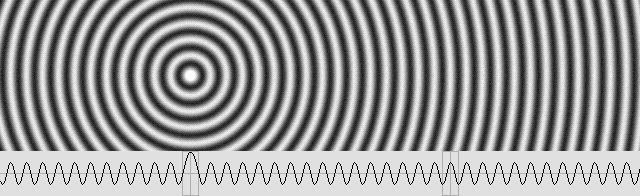The electron and the positron phase oscillate according to a quarter of period offset.

Firstly, the wave addition produces standing waves, especially along the axis.

Secondly, the gluonic field is amplified into a quark in such a way that the particles seem much weaker.

Energy is linked to the speed of light squared.

It was shown that a 1 kg mass gain is equivalent to the energy given by the famous mc squared formula.

E  =  c 2

E  =  9 * 10 16  joules.

According to our example, it is the amount of kinetic energy which is stocked inside a billiard ball whose mass at rest was 1 kg but whose speed is now 0.866 c. Quite certainly, the two billiard balls would be destroyed at such a speed, but it is not the point.

This new way of seeing the process definitely confirms that the famous mc squared equation is highly relevant. This is no surprise, but it is about time that scientists realize that energy is strongly dependant on the speed of light. Matter behaves this way because it contains waves whose speed is that of the light. This constant speed is actually that of all aether waves, including the light and matter itself.

More examples.

If you doubt this, below is an equation which systematically yields a one kilogram gain in mass whatever the mass at rest. Then Lorentz's contraction factor is given by:

g  =  m / (m + 1)

For example, Lorentz's factor is 0.2 for a 0,25 kg stationary mass. Because the mass is increased to: M  =  1.25 kg, the mass gain is indeed one kilogram exactly and in this case, the beta normalized speed is given by:

beta  =  (1 – g 2) 1 / 2

beta  =  0.979795897

v  =  beta  =  293938769 m/s        m  =  0.25 kg        g  =  0.2

E  =  c 2  =  0.25 v 2 / (0.2 2 + 0.2)  =  9 * 10 16  joules.

Henri Poincare was a pioneer.

Let's compare the mass for stationary matter to the gain in mass as kinetic energy. We are in the presence of two apparently separate entities which nevertheless are present in the same object and share the same properties. Energy for both of them is given by the same m c squared equation. What's more, their behavior is clearly linked to the Doppler effect: this was demonstrated in the previous page on active and reactive mass. All this strongly suggests that we are in the presence of waves, and that those two mass entities could actually be the same wave system simply growing up.

Standing waves responsible for stationary matter, which contain intrinsic energy according to mc^2, may additionally contain potential energy. This occurs when the system is no longer stationary as a result of the wave compression, which is a mere consequence of the Doppler effect. Moreover, inertia is a resistance to a force, which produces an acceleration according to f = m*a. This is quite possible solely in the presence of waves. All standing waves contain energy. In the presence of another standing wave set, the wave addition and the subsequent amplification by more aether waves traveling in the vicinity surely can exert a radiation pressure. This scheme indicates that all other aether waves and especially the light also contain energy. Thus, they may also be considered as mass.

Poincare was unaware of this as a whole, but he nevertheless thought that the light energy could indeed be compared to mass. He found the equivalent of the famous m*c^2 equation before Einstein. It becomes clear that his formula below is strangely relevant and that it must definitely be considered as a giant step:

m = E / c 2

And obviously:

E = m c 2

Mr. Jules Leveugle reports that as soon as 1900, Henri Poincare established that mass (and the corresponding inertia and energy) could be one of the properties of light and that he then released this formula. He was thus well in advance as compared to Einstein.

Unfortunately, Poincare was absolutely convinced that he was dealing with "electromagnetic waves". Such waves do not exist. The light, including all radio waves, is rather made of regular aether waves. Such waves are responsible for all action and reaction forces. I could not examine Poincare's document because I live in North America, not in France. Some of his works are not easily available because Poincare was not allowed to publish them in most French scientific publications. Although I ignore how he came to this conclusion, I am quite sure that his reasoning was as good as that of Einstein.

Einstein only established the corollary, that is to say the reaction that a material body would oppose to a given quantity of emitted light. His attitude was unfair because he never quoted Poincare. Most scientists consider that Einstein found the famous equation alone. However, it is a well known fact that he was fully aware of Poincare's works. So there is no place for admiration here: on the contrary, it is a shame.

The mass gain according to Poincare.

Mr. Serge Cabala reveals interesting information about what was going on in the scientific world around 1900. The important point is that Poincare's analysis was received in Palermo (French editors were not available!) in July 1905 but that he had already transmitted a note to the French Academy of Science on June 5, 1905, hence before the publication of Einstein's Special Relativity in Sept. 1905.

Lorentz predicted that matter should undergo a greater inertia at high speed. This was well verified as soon as 1904, but the electron was a special case because of its electric charge. Its inertia proved to be different axially and transversally. However, in the case of matter, Poincare found that the mass gain should be given by:

E  =  gamma * m c 2

Obviously, when matter is stationary, the gamma factor is 1 and it can be removed from the equation. Then one obtains again the famous equation:

E = m c 2

Active and reactive forces.

From an absolute point of view, hence according to Lorentz, action and reaction effects are not equal because of the Doppler effect. All fields of force contain energy which is radiated toward matter in opposite directions. All aether waves can exert a radiation pressure on matter. Before examining Newton's laws, one must be aware that this basic wave mechanism is subject to Lorentz's Doppler effect. The more a wave is compressed, the more the force is powerful. I must repeat again that this was demonstrated in the previous page on active and reactive mass.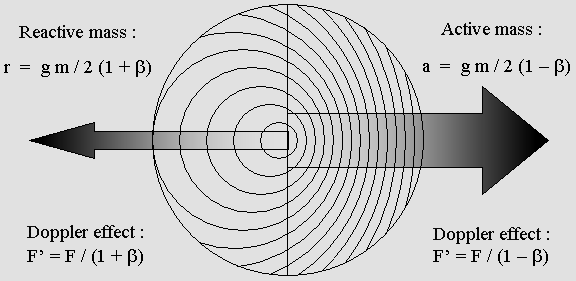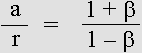Action and reaction forces are unequal because they are caused by waves undergoing the Doppler effect.

Because kinetic energy is not a mystery any more, we are now ready to upgrade Newton's laws on matter mechanics.

NEWTON'S LAWS ON MOTION REVISITED

Newton's laws prove to be fairly accurate most of the time. They are still in usage today, probably because Einstein's mechanics is horribly complex. Even engineers working for NASA or on the GPS system are very uncomfortable with Einstein. Unfortunately, fast moving matter no longer behave like Newton predicted. The chief cause of the error is that the wave properties of matter had not yet been discovered. The Doppler effect leads to more and more energy as a consequence of the wave compression. Inertia proves to be progressive in such a way that the speed of light is unreachable for matter. Poincare was well aware of such a progressive inertia as soon as 1904:

 "We will perhaps need to invent some new mechanics that we can hardly foresee, where inertia increases with the speed in such a way that the speed of light would be an insuperable limit."

Henri Poincare's "new mechanics".

Lorentz and Poincare already constructed a large part of the building, but because of the wave nature of matter it desperately needs a new cornerstone. The goal here is to obtain more accurate results, but starting from scratch by totally replacing Newton's laws appears quite a challenge. Revisiting Lorentz's Relativity and Newton's laws is a better option because they are much more understandable than Einstein's Relativity. In addition, they are compatible with Euclidean geometry.

Newton's mechanics is upgradeable,

So let's upgrade Newton's laws. This is only a first try, but I am quite sure that they will some day become perfectly accurate and still easy to understand in spite of the fact that the absolute version of Lorentz's Relativity indicates that they are totally false. The point is that the absolute truth will always remain hidden. Mainly because of the Doppler effect, we are dealing with appearances. We have no choice: we must accept Relativity.

We have to deal with variable mass and with the Lorentz transformations. Those new behaviors must be integrated to Newton's mechanics in order to obtain the more accurate new mechanics. Then all phenomena will seem to occur as predicted, from a relative point of view. This point of view must also be chosen carefully. It should be a preferred frame of reference in order to avoid paradoxes (not to say contradictions) which are common in Einstein's version.

Newton's second law is especially confusing because it indicates that a force must be applied to a material body in order to change its velocity or direction. As long as no force is applied, it is much simpler to consider that this body is stationary. In this case, the Lorentz transformations do not apply and the mass is simply the mass at rest. It is already well admitted that the center of inertia, even for multiple material bodies undergoing action and reaction forces, cannot undergo any direction or speed variation. Finally, only the speed difference must be applied separately to those bodies and the Lorentz transformations are not to be taken into account if this difference is not important. In this case, one should consider that Newton's laws are already correct. If the speed difference appears important (relativistic speed), it is still possible to apply the mass gain and the Lorentz transformations.

The preferred frame of reference.

Two different formulas for kinetic energy are now available. Poincare's one is absolute because the frame of reference is postulated to be stationary with respect to the aether. The revisited Newtonian one is rather relative to the observer's frame of reference, which must however be chosen carefully. Surprisingly, they both yield accurate results. This is quite comforting as compared to Einstein's Relativity, which was rather plagued with paradoxes which could easily be interpreted as contradictions. For example, two observers whose speed differs always see "the other one" being contracted. This annoying reciprocity should be avoidable. The situation is even worse if more than two observers moving at different speeds are present. The choice of an acceptable frame of reference as a convention suddenly appears crucial.

The next page on fields of force explains that those fields exert a radiation pressure in two opposite directions. The forward and backward force is proportional to the wave contraction or dilation in accordance with the Doppler effect. However, it always appear equal on both sides on condition that the field of force is postulated to be stationary at the center of inertia of the system. Then Newton's equal and opposite action and reaction law holds true. The important point is that the center of inertia may easily be considered constantly at rest because interactions between many material bodies do not change its status. It is the perfect place for a truly impartial and reliable arbitrator. This leads to much simpler calculus because Relativity (even Lorentzian) is no longer to be considered. Let's be honest: nobody could really deal with Relativity, including Einstein himself! However, the gain in mass and the Lorentz transformations still apply.

We are aware that Newton's original mechanics works well on condition that the speed is reasonably slow. It is now possible to upgrade it. It will be perfectly accurate at any speed under three conditions:

1. - The center of inertia as a preferred frame of reference should be identified.

2. - The mass gain must be taken into account.

3. - Mater transforms according to Lorentz's equations.

This should make us happy. We definitely prefer avoiding relativistic effects, such as trying to see how the world would look like if we were following an electron inside a large collider at nearly the speed of light. We can observe two electrons traveling in opposite directions colliding at nearly two times the speed of light. This is true as seen from the collider, but not as seen in a fast moving frame of reference because any speed faster than the speed of light is impossible. It is definitely easier to rely on Newton's laws.

Let's make it simple.

We definitely prefer avoiding a complex tensorial calculus in order to obtain the precession of the perihelion of Mercury's orbit. Almost nobody on this planet could obtain the correct result, especially because all other planets are involved and also because the correct frame of reference should also be considered. As a matter of fact, considering the whole solar system, the sun itself is rotating around a center of inertia. And this idea of bending space is totally absurd. Let's face it: Einstein's method is quite weird.

Gravity does not obey exactly Newton's equation for Mercury's short distance to the sun. This occurs for the same reason that there is no gravity at all in the center of the sun while the formula yields an infinite result. Near to the sun, some of its mass exerts a gravity force from an angle. Thus, the gravity curve is bending there much the same way Ricci tensors do. It is very easy to arbitrarily make them reach the point which produces the correct perihelia precession. Frankly, the so-called "error" is frankly negligible and does not invalidate Newton's equation. It just indicates that other anomalies are present and that they should be identified.

Let's be clear: gravity is just an ordinary force. Basically, it obeys Newton's equation, albeit some situations need additional correction. In a very fast moving frame of reference, all forces are submitted to relativistic effects. Not just gravity. Surely, establishing a complex, hard to manage "General Relativity" especially for gravity was totally ridiculous and unnecessary.

We need a more simple, universal method in order to obtain more accurate results, and revisiting Newton's laws of motion appears to be the best one.

The field of force.

Energy is the effect or the cause of a force which is applied during a given period of time or along a given distance. It may produce an acceleration or a deceleration, and it can also change the direction. Matter velocity is well known to be kinetic energy, but potential energy as a material field of force is not yet well accepted. The important point is that a field of force is capable of accelerating matter thanks to the radiation pressure. The best example is the electrostatic field of force shown above.

Two electrons or two positrons produce a repulsive field of force while an electron and a positron rather produce an attractive field of force. In both cases, the result is an acceleration, hence kinetic energy.

This occurs because electrons or positrons are constantly pulsating spherical waves. The space between two of them is automatically filled with standing waves, which are responsible for the Coulomb force

Those standing waves are amplified by all aether waves in the vicinity, the same way electrons are. The more they are close together, the more they contain energy. This energy is returned toward both particles and the phase determines whether the effect will be attraction or repulsion.

I was able to show recently that, on condition that the medium is compressible (such as air), standing waves are capable of modifying the speed of waves traveling along the axis. This phenomenon will be easily verifiable using acoustic devices. It becomes clear that waves may exhibit variable behaviors depending on the medium properties. They definitely have a "personality". For example, larger waves on water travel much faster than small waves. Yet, most physicists still rely on equations to study waves. This attitude hides the fact that we are dealing with a rather complex and surprising phenomenon. The main conclusion is that spherical standing waves are capable of interaction.

An electrostatic  field of force does not contain any electron or positron. Except for its effects, it is quite difficult to detect as a place containing significant amounts of energy. It may be considered as matter according to the famous m c squared equation, especially when electrons and positron come very close together. Then the field of force progressively transforms into a gluonic field, whose energy is much greater than that of an isolated electron. I strongly believe (but it is still to be demonstrated!) that this system, which contains an electron, a positron and a gluonic field of force, should be a quark. Three quarks placed crosswise, one being different in structure, should (possibly!) produce a neutron.

From a mechanical point of view, one may consider that the field of force is located exactly at the center of inertia, which is in the middle of the axis joining the electrons or the positrons. The mass of all electrons being the same, it becomes clear that its force should apply equally on both electrons, especially if the center of inertia and the observer are postulated to be stationary.

The Double Action Principle.

Any attraction or repulsion effect is a consequence of the radiation pressure, which is applied equally in opposite directions by the field of force. This suggests Newton's action and reaction law, but in this case the center of inertia does not move. If it were moving, action and reaction would not be equal any more because the Doppler effect would produce waves whose wavelength differs forward and backward. The result is unequal active and reactive forces incoming from active and reactive mass, which is present inside the field of force.

If the mass of two material bodies is not the same, the acceleration is faster for the smaller body. As a result, the center of inertia remains stationary. However, in the case of gravity between the moon and the earth, for example, the fields of force are repulsive fields which are placed beyond each of them. The moon seems attracted, but it is actually pushed towards the earth. A "virtual" attractive field seems to be placed exactly in the middle, not at the center of inertia. The result is a simultaneous action which neutralizes a possible torque effect. Many authors had noted that gravity should be transmitted instantaneously (not at the speed of light) in order to avoid such a torque effect.

I - Newton's first law: the Inertia Principle.

The frame of reference of an isolated material body is obviously the one where it seems stationary. One could hardly think that its frame of reference is the one where it seems to move at a constant speed and along a straight line. This is what Newton seems to admit, though. If it seems to move, the frame of reference is rather that of an observer whose speed is not the same. Such an idea could be acceptable according to Galileo's Relativity Principle, but it is now totally obsolete because of the Lorentz transformations. Today, the only acceptable frame of reference is Cartesian, not Galilean, and it must be postulated to be stationary.

This preferred, if not unique Cartesian frame of reference should theoretically be at rest as compared to the aether. However, Lorentz and Poincare also discovered that such a reference is unverifiable. The only remaining compromise is to simply postulate that a given frame of reference is stationary. This choice is indeed possible according to Relativity. If a material body is moving (or if it seems to be moving) inside this frame of reference, one must consider that it is undergoing the Lorentz Transformations, more exactly the Doppler effect. Lorentz's equations or my Time Scanner can finally show how it should behave.

In brief, one cannot consider a moving frame of reference unless everything inside it is undergoing the Lorentz transformations. The only easy way to observe the moving equivalent under the Lorentz transformations is to submit it to my Time Scanner. For example, a rotating gear should look like this:The center of the gear on the left hand side is stationary but the external part is rotating at 86.6% of the speed of light.

The equivalent gear shown on the right was accelerated by the Time Scanner to 86.6% of the speed of light.

Because matter cannot reach the speed of light, the upper part moves to the right at 0.9897 c only.

Please note that all moving parts are undergoing a contraction according to Lorentz's shrinking factor.

The center of inertia appears to be the best place for establishing the frame of reference. It is especially useful in the presence of multiple bodies undergoing interaction because it never moves. For instance, the center of inertia of the solar system remains at rest and the sun rather rotates around it because it is attracted mainly by Jupiter and Saturn, especially when they come close together. This is a good basis for the next page on Fields of Force Dynamics.

That is why Newton's first law needs to be worded differently, possibly this way:

 The center of inertia is postulated to remain stationary in its frame of reference.

Newton's First Law revisited.

This preferred frame of reference is also that of the observer, who is postulated to be stationary as well.

It should be pointed out that Newton admitted that the Inertia Principle was Galileo's discovery.

Formerly:

"Every object in a state of uniform motion tends to remain in that state of motion unless an external force is applied to it."

II - Newton's second law: Force and Acceleration.

The well-known formula:  f = m*a  should apparently become inaccurate at very high speed because of the Doppler effect, which causes the mass to progressively build up according to the gamma factor. However, acceleration decreases while mass increases and surprisingly, the original formula seems to remain correct. An elaborated analysis using active and reactive mass should confirm this. The notion of acceleration will also need to be revisited.

Once again, the observer must be postulated to be stationary in order to avoid contradictory results. As Newton pointed out, one must take the composition of forces (which behave according to Pythagoras' theorem) into account. But in addition, because Newton was unaware that matter inertia, energy and mass are variable, I propose that his second law on force and acceleration should be upgraded as follows:

 Considering that its mass increases according to Lorentz's gamma factor as seen from a frame of reference which is postulated to be stationary, the acceleration of a material body  is proportional to the force acting on it and inversely proportional to its mass.

Newton's Second Law revisited.

At high speed, the Doppler effect causes matter mass to increase proportionally to the gamma factor.

That is why the body accelerates less and less rapidly if the applied force is constant.

Formerly:

"The acceleration of an object is proportional to the force acting on it and inversely proportional to its mass."

III - Newton's third law: the stationary field of force generates two equal and opposite actions.

The law of conservation of mass and energy always holds true. As seen from a stationary frame of reference which is not that of the center of inertia, the total amount of mass T shown below never changes: T = 3 kg. However, another observer placed in the frame of reference of the other ball would obtain the reversed contradictory version.The frame of reference is that of the ball on the right hand side before the collision.

The force may be applied by any field of force, for example a magnetic field.

Delta stands for mass which is temporarily stored into the field of force while the force is applied.

It was found around 1900 that the light could exert a radiation pressure on a material body and that the pressure is stronger if the light is reflected. This suggests that there is an action and reaction effect and that a laser should be pushed backward while it is emitting a light beam, much the same way a cannon is. But obviously, the light could not push a material body faster than the speed of light. The field of force could not emit a radiation beam towards the billiards ball either. That is why energy or kinetic energy, whose amount is given by the m c squared formula, is linked to the speed of light "c".

In the animated gif shown above, a problem arises because the frame of reference is not that of the center of inertia. The asymmetry introduces a more complex calculus whose results are not consistent as seen from any other frame of reference. Fortunately, if the frame of reference is postulated to remain at the center of inertia, the results become symmetric and they are less likely to appear different anywhere else. The "double action" collision below is definitely more acceptable.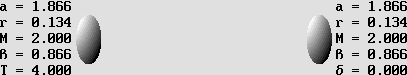The frame of reference is that of the center of inertia.

Now, the collision phenomenon is much easier to understand and to deal with.

The transposition is not equivalent here because the total mass M is 4 kg instead of 3. If just one ball was considered to be moving, its speed should be 0.9428 c and its mass, 3 kg. From an absolute point of view, if the speed is unequal, action and reaction are also unequal because of the Doppler effect. However, this point of view must be ruled out because of Relativity. The only remaining option (the only interesting one, actually) is to consider that the field of force is stationary and that it radiates exactly the same amount of energy on both sides. Thus, there is no specific action or reaction any more. One must rather consider that the field of force exerts exactly the same force in two opposite directions, that is on the two billiard balls located on each side of the center of inertia. This might be called the "Double Action Principle".

 The field of force which is postulated to be located at the center of inertia of two material bodies exerts an equal and opposite action on both of them in such a way that it remains stationary.

Newton's Third Law revisited.

Double Action replaces Action and Reaction.

This phenomenon is developed in the next page on the fields of force dynamics.

Formerly:

"For every action there is an equal and opposite reaction."

In the presence of multiple bodies, the center of inertia of the whole system is still the best place for establishing the frame of reference from where the observer will have a better view. The composition of a large number of fields of force will have to be considered. Fortunately, most of the time, it remains possible to obtain fairly good results using the Double Action Principle. But when relativistic speeds must be taken into account, the forces become unequal. Active and reactive forces appear. As seen from the center of inertia, they are proportional to the forward and backward Doppler effect. Thus, a fairly accurate calculus for the solar system using the Double Action Principle appears feasible, but a highly accurate calculus using active and reactive forces would be much more complicated to perform.

The Fields of Force Dynamics.

The dynamics of matter is all about wave dynamics. However, for practical reasons, one should rather name it the fields of force dynamics. The important point is that the Lorentz transformations and Relativity must be taken into account in order to upgrade Newton's laws on motion.

Einstein's Theory of Relativity is very annoying to deal with because it doesn't appear logical. Especially, it always yields two different and reciprocal results. For example, two observers whose speed is not the same always see "the other one" undergoing a contraction. One should admit that this reciprocity is not a "paradox". Let's face it, it is purely a contradiction which indicates that one of them at least (and more likely both of them) is wrong because he is fooled by the Doppler effect.

The preferred frame of reference.

The good news is that the composite field of force responsible for interactions between two (or many) material bodies is located at the center of inertia, which may most of the time be postulated to be stationary. The point is that it does not move whatever the result of the interaction. This is the perfect place to establish a preferred frame of reference where an observer will not be fooled by the Doppler effect. From this point of view, the situation is no longer symmetrical because it is unique. All other observers should admit that this place is indeed a reliable one to establish the Cartesian axes. They have no choice anyway: it is the unavoidable condition under which Newton's laws on motion hold true.

On the one hand, the observer placed at the center of inertia is impartial because he doesn't have any reason to prefer any other situation. His frame of reference is the best one, it is unique, and as seen from this place there is no Relativity any more. His only remaining task is to establish the amount of transformation of all other moving bodies according to Lorentz's equations. My Time Scanner can also perform this operation.

On the other hand, this impartial observer certainly doesn't see what is truly going on. Lorentz and Poincare discovered that the only one and absolute situation will remain unknown forever.

This indicates that, most certainly, action and reaction are unequal from an absolute point of view. They are proportional to action and reaction forces as a result of the Doppler effect. Moreover, they are not simultaneous. Newton's laws on motion being revisited, let's examine why and how causes produce effects.

A new Causality Principle.

It is true that every effect has a cause. But today, it becomes obvious that this effect is a motion and that the cause which produced this motion was a wave traveling through the aether at the speed of light. Motion appears to be the result of a modification of forward and backward matter wavelength according to the Doppler effect. Lorentz discovered that there is no wavelength modification transversally: y'=y; z'=z. This singularity (the regular Doppler effect introduces a wavelength contraction transversally) supposes that the electron frequency slows down according to Lorentz's contraction factor.

It turns out that the process is strongly dependant on the speed of light, which introduces a delay between a cause and its effect.

The important point is that effects transform into new causes, which then produce new effects. This endless chain reaction is the basis of matter mechanics and we have shown that, quite surprisingly, a mechanical wave propagates much the same way.

So, in that order, we have a motion, a stronger compressed emitted wave because of the Doppler effect, a field of force when this wave encounters another one incoming from another material body, a radiation pressure generated by the field of force towards this body, and a motion again. As seen at the electron scale, the splendor of our universe is nothing but the sum of motions generating more motions.

Thus, a new Causality Principle might be worded as follows:

 Any effect has a cause, and this effect produces new causes which are transmitted by waves at the speed of light.

A new Causality Principle.

Because causes are always different, it appears unlikely to be possible that the "the same causes always produce the same effects". Such a statement introduces a serious problem about determinism. The future might be the inexorable sum of all of today's causes, but this is unverifiable.

Around 1900, during the period preceding the discovery of Relativity, scientists were divided. They could not reach a consensus about a mechanist or a probabilistic approach. The probabilistic choice could rule out the hard to conceive determinism. Statistics prove to be quite logic, and most randomly distributed events sometimes end up with surprising coincidences. But a mechanism leading to liberty appears improbable. If Newton's laws are unavoidable, the mechanistic choice is the only logic one.

Our situation could be that of passengers traveling in a train wagon. Considering that the railroad is already in place and that it leads to some unknown destination, which is however already well established, we can merely observe the landscape and wait until our fate is accomplished. However, most people categorically reject this philosophy.

To be honest, the ultimate question should rather be: what was the Cause of the aether?

The speed of light is constant and decisive.

Any radiation is transmitted through the aether at the speed of light. Because energy decreases according to the square of the distance law, the same radiation cannot produce the same effect everywhere simultaneously and with the same force.

In addition, because of the Lorentz transformations, a moving phenomenon is (or seems) severely distorted. Because of the Doppler effect, the waves traveling forward are slower and the mechanical result is a local time, which is given by Lorentz's t' second equation. In addition, the speed of light traveling in a moving system is slower, even from an absolute point of view, because the light path is longer, especially axially. This was discovered by Michelson. Thus, forces are also distorted proportionally and matter mechanics as well. Surely, Lorentzian Relativity appears much more reasonable today, and it strongly suggests that matter is made of waves.

It becomes clear that Newton's laws on motion are strongly related to the speed of light in a way that Newton never suspected. The famous m*c squared equation must definitely be integrated to Newton's mechanics, considering that the speed of light "c" is constant with respect to the aether.

Surely, Einstein's postulate stating that the speed of light is the same in all Galilean frames of reference is wrong.

Gravity does not bend space.

A gravitational field of force seems present between two material bodies. It apparently attracts them from an arbitrary center of inertia. But actually, gravity is caused by at least four fields of force, whose component finally pushes them towards each other.

Let's be clear: gravity is not linked to Relativity. Elaborating a complex "General Relativity" in order to just explain gravity was a mistake. Gravity is much more simply explainable thanks to the fields of force dynamics. This method proposes the center of inertia as a preferred frame of reference. The interesting point is that it allows one to consider that this place is no longer submitted to Relativistic effects. What's more, Newton's formula on gravity can be recovered without any modification, on condition that any moving material body is postulated to be submitted to the Lorentz Transformation according to its speed as it is seen from this frame of reference. This includes the gain in mass, which is responsible for kinetic energy.

Frankly, gravity cannot bend space. This is ridiculous.

Newton's glorious work is now accurate for Eternity.

The important point to remember is that Newton was unaware that matter waves are undergoing the Doppler effect, more exactly the Lorentz Transformations. Because the goal is to upgrade Newton's mechanics, the obvious conclusion to draw is that one must now take the Lorentz transformations into account. This should fix the problem forever.

Because it is all about integrating the Doppler effect to Newton's discoveries, the page on active and reactive mass appears of the utmost importance. Lorentz predicted that in a moving system, any periodic phenomenon such a an emitter frequency should slow down. For example, assuming that beta is 0.866, the electron frequency would be reduced to only 50% of its original frequency:

F '  =  g F  =  0.5 F

The basic frequency being slower, the forward absolute wavelength still contracts and the force applied forward becomes stronger. The wave energy (hence matter mass) being proportional to the frequency, it also increases. Active force "a" is given by:

Fa  =  F ' / (1 – beta)  =  0.5 / (1 0.866)  =  3.732 F

The force exerted by waves traveling forward or backward is represented by a and r, which stand for action and reaction. Here, the force exerted forward is 3.732 times that of a stationary electron. The force exerted backward is rather given by:

Fr  =  F ' / (1 + beta)  =  0,5 / (1 + 0.866)  =  0.26795 F

It should be emphasized that, unlike the regular Doppler effect, this "relativistic" Doppler effect is perfectly symmetrical. One force is exactly the inverse (1 / x) of the other. That is why Lorentz's Relativity becomes possible.

Fa * Fr = 1

1 / Fa  =  Fr

1 / 3.732  =  0.26795

Kinetic energy according to the gamma factor is just a consequence of Lorentz's slower Doppler effect. Knowing this, Newton's work becomes logical, comprehensive and accurate. I am well aware that this page needs a severe revision, but its basic principles cannot be doubted any more because the wave mechanics always lead to correct predictions.

Let's face it: Einstein did not explain why and how those phenomena are possible.

Fortunately, it was not that complicated.

| 01 | 02 | 03 | 04 | 05 | 06 | 07 | 08 | 09 | 10 | 11 | 12 | 13 | 14 | 15 | 16 |

| You are here | 18 | 19 | 20 | 21 | 22 | 23 | 24 | 25 | 26 | 27 | 28 | 29 | 30 | 31 | 32 | 33 |

 Gabriel LaFreniere Bois-des-Filion in Québec. On the Internet since September 2002. This page was translated from French in June 2009. Updated December 3, 2009.